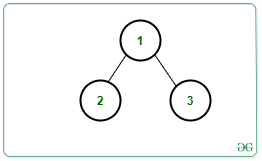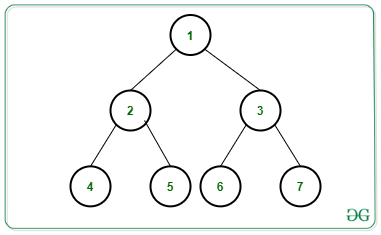# Count root to leaf paths having exactly K distinct nodes in a Binary Tree

• Difficulty Level : Hard
• Last Updated : 22 Jun, 2021

Given a Binary Tree consisting of N nodes rooted at 1, an integer K and an array arr[] consisting of values assigned to each node, the task is to count the number of root to leaf paths having exactly K distinct nodes in the given Binary Tree.

Examples:

Input: N = 3, Edges[][] = {{1, 2}, {1, 3}}, arr[] = {3, 3, 2}, K = 2, Below is the given Tree:Output: 1
Explanation:
There exists only 1 distinct path i.e., Path 1 -> 3 contains 2 distinct nodes.

Input: N = 7, Edges[][] = {{1, 2}, {1, 3}, {2, 4}, {2, 5}, {3, 6}, {3, 7}}, arr[] = {2, 2, 2, 2, 3, 5, 2}, K = 1, Below is the given Tree:Output: 2
Explanation:
There exists only 2 distinct paths containing 1 distinct node:
1) Paths 1 -> 2 -> 4,
2) Paths 1 -> 3 -> 7

Naive Approach: The simplest approach is to generate all possible paths from the root to the leaf nodes and for each path, check if it contains K distinct nodes or not. Finally, print the count of such paths.

Time Complexity: O(N * H2), where H denotes the height of the tree.
Auxiliary Space: O(N);

Efficient Approach: The idea is to use Preorder Traversal and a Map to count the distinct node in the path from the root to the current node. Follow the below steps to solve the problem:

• Initialize a variable distinct_nodes as 0 to store the count of the distinct node from the root to the current node and ans as 0 to store the total distinct root to leaf paths having K distinct node.
• Perform Preorder Traversal in the given binary tree and store the count of the distinct node from root to the current node in the map M.
• Whenever a node occurs for the first time on a path, increase the count of distinct nodes by 1.
• If the count of distinct nodes on a path becomes greater than K return to the parent node of the current node.
• Otherwise, continue to visit the children of the current node incrementing the frequency of the current node value by 1.
• In the above step, increment ans by 1 if the count of distinct nodes on that root to leaf path is exactly equal to K.
• After the above steps print the value of ans as the resultant count.

Below is the implementation of the above approach:

## C++

 `// C++ program for the above approach` `#include ` `using` `namespace` `std;`   `// Structure of a Tree Node` `struct` `Node {` `    ``int` `key;` `    ``Node *left, *right;` `};`   `// Function to create new tree node` `Node* newNode(``int` `key)` `{` `    ``Node* temp = ``new` `Node;` `    ``temp->key = key;` `    ``temp->left = temp->right = NULL;` `    ``return` `temp;` `}`   `// Function to count all root to leaf` `// paths having K distinct nodes` `void` `findkDistinctNodePaths(` `    ``Node* root, unordered_map<``int``, ``int``> freq,` `    ``int` `distinct_nodes, ``int` `k, ``int``& ans)` `{` `    ``// If current node is null` `    ``if` `(root == NULL)` `        ``return``;`   `    ``// Update count of distinct nodes` `    ``if` `(freq[root->key] == 0)` `        ``distinct_nodes++;`   `    ``// If count > k then return to` `    ``// the parent node` `    ``if` `(distinct_nodes > k)` `        ``return``;`   `    ``// Update frequency of current node` `    ``freq[root->key]++;`   `    ``// Go to the left subtree` `    ``findkDistinctNodePaths(root->left,` `                           ``freq,` `                           ``distinct_nodes,` `                           ``k, ans);`   `    ``// Go to the right subtree` `    ``findkDistinctNodePaths(root->right,` `                           ``freq,` `                           ``distinct_nodes,` `                           ``k, ans);`   `    ``// If current node is leaf node` `    ``if` `(root->left == NULL` `        ``&& root->right == NULL) {`   `        ``// If count of distinct node` `        ``// is same as K, increment ans` `        ``if` `(distinct_nodes == k)` `            ``ans++;` `    ``}` `}`   `// Function to find count of root to` `// leaf paths having K distinct node` `void` `printkDistinctNodePaths(Node* root,` `                             ``int` `k)` `{` `    ``// Initialize unordered map` `    ``unordered_map<``int``, ``int``> freq;`   `    ``// Stores count of distinct node` `    ``int` `distinct_nodes = 0;`   `    ``// Stores total count of nodes` `    ``int` `ans = 0;`   `    ``// Perform Preorder Traversal` `    ``findkDistinctNodePaths(root, freq,` `                           ``distinct_nodes,` `                           ``k, ans);`   `    ``// Print the final count` `    ``cout << ans;` `}`   `// Driver Code` `int` `main()` `{` `    ``/*         2` `             ``/   \` `            ``/     \` `           ``1       3` `          ``/ \     /  \` `         ``/   \   /    \` `        ``4     2 -5     3` `    ``*/`   `    ``// Given Binary Tree` `    ``Node* root = newNode(2);` `    ``root->left = newNode(1);` `    ``root->right = newNode(3);` `    ``root->left->left = newNode(4);` `    ``root->left->right = newNode(2);` `    ``root->right->left = newNode(-5);` `    ``root->right->right = newNode(3);`   `    ``// Given K` `    ``int` `K = 2;`   `    ``// Function Call` `    ``printkDistinctNodePaths(root, K);`   `    ``return` `0;` `}`

## Java

 `// Java program for the ` `// above approach` `import` `java.util.*;` `class` `GFG{`   `// Structure of a ` `// Tree Node` `static` `class` `Node ` `{` `  ``int` `key;` `  ``Node left, right;` `};` `  `  `static` `int` `ans;` `  `  `// Function to create ` `// new tree node` `static` `Node newNode(``int` `key)` `{` `  ``Node temp = ``new` `Node();` `  ``temp.key = key;` `  ``temp.left = temp.right = ``null``;` `  ``return` `temp;` `}`   `// Function to count all root ` `// to leaf paths having K ` `// distinct nodes` `static` `void` `findkDistinctNodePaths(Node root, ` `                                   ``HashMap freq, ` `                                   ``int` `distinct_nodes, ` `                                   ``int` `k)` `{` `  ``// If current node is null` `  ``if` `(root == ``null``)` `    ``return``;`   `  ``// Update count of distinct nodes` `  ``if` `(!freq.containsKey(root.key))` `    ``distinct_nodes++;`   `  ``// If count > k then return ` `  ``// to the parent node` `  ``if` `(distinct_nodes > k)` `    ``return``;`   `  ``// Update frequency of ` `  ``// current node` `  ``if``(freq.containsKey(root.key))` `  ``{` `    ``freq.put(root.key, ` `    ``freq.get(root.key) + ``1``);` `  ``}` `  ``else` `  ``{` `    ``freq.put(root.key, ``1``);` `  ``}`   `  ``// Go to the left subtree` `  ``findkDistinctNodePaths(root.left, freq,` `                         ``distinct_nodes, k);`   `  ``// Go to the right subtree` `  ``findkDistinctNodePaths(root.right, freq,` `                         ``distinct_nodes, k);`   `  ``// If current node is ` `  ``// leaf node` `  ``if` `(root.left == ``null` `&& ` `      ``root.right == ``null``) ` `  ``{` `    ``// If count of distinct node` `    ``// is same as K, increment ans` `    ``if` `(distinct_nodes == k)` `      ``ans++;` `  ``}` `}`   `// Function to find count of root to` `// leaf paths having K distinct node` `static` `void` `printkDistinctNodePaths(Node root, ` `                                    ``int` `k)` `{` `  ``// Initialize unordered map` `  ``HashMap freq = ``new` `HashMap<>();`   `  ``// Stores count of ` `  ``// distinct node` `  ``int` `distinct_nodes = ``0``;`   `  ``// Stores total ` `  ``// count of nodes` `  ``ans = ``0``;`   `  ``// Perform Preorder Traversal` `  ``findkDistinctNodePaths(root, freq,` `                         ``distinct_nodes, k);`   `  ``// Print the final count` `  ``System.out.print(ans);` `}`   `// Driver Code` `public` `static` `void` `main(String[] args)` `{` `  ``/*           2` `             ``/   \` `            ``/     \` `           ``1       3` `          ``/ \     /  \` `         ``/   \   /    \` `        ``4     2 -5     3` `    ``*/`   `  ``// Given Binary Tree` `  ``Node root = newNode(``2``);` `  ``root.left = newNode(``1``);` `  ``root.right = newNode(``3``);` `  ``root.left.left = newNode(``4``);` `  ``root.left.right = newNode(``2``);` `  ``root.right.left = newNode(-``5``);` `  ``root.right.right = newNode(``3``);`   `  ``// Given K` `  ``int` `K = ``2``;`   `  ``// Function Call` `  ``printkDistinctNodePaths(root, K);` `}` `}`   `// This code is contributed by gauravrajput1`

## Python3

 `# Python3 program for the above approach`   `# Structure of a Tree Node` `class` `newNode:` `    `  `    ``def` `__init__(``self``, key):` `        `  `        ``self``.key ``=` `key` `        ``self``.left ``=` `None` `        ``self``.right ``=` `None`   `ans ``=` `0`   `# Function to count all root to leaf` `# paths having K distinct nodes` `def` `findkDistinctNodePaths(root, freq, ` `                           ``distinct_nodes, k):` `                               `  `    ``global` `ans` `    `  `    ``# If current node is None` `    ``if` `(root ``=``=` `None``):` `        ``return`   `    ``# Update count of distinct nodes` `    ``if` `(root.key ``not` `in` `freq):` `        ``distinct_nodes ``+``=` `1`   `    ``# If count > k then return to` `    ``# the parent node` `    ``if` `(distinct_nodes > k):` `        ``return`   `    ``# Update frequency of current node` `    ``if` `(root.key ``in` `freq):` `        ``freq[root.key] ``+``=` `1` `    ``else``:` `        ``freq[root.key] ``=` `freq.get(root.key, ``0``) ``+` `1`   `    ``# Go to the left subtree` `    ``findkDistinctNodePaths(root.left, freq,` `                           ``distinct_nodes, k)`   `    ``# Go to the right subtree` `    ``findkDistinctNodePaths(root.right, freq,` `                           ``distinct_nodes, k)`   `    ``# If current node is leaf node` `    ``if` `(root.left ``=``=` `None` `and` `       ``root.right ``=``=` `None``):` `        `  `        ``# If count of distinct node` `        ``# is same as K, increment ans` `        ``if` `(distinct_nodes ``=``=` `k):` `            ``ans ``+``=` `1`   `# Function to find count of root to` `# leaf paths having K distinct node` `def` `printkDistinctNodePaths(root, k):` `    `  `    ``global` `ans` `    `  `    ``# Initialize unordered map` `    ``freq ``=` `{}`   `    ``# Stores count of distinct node` `    ``distinct_nodes ``=` `0`   `    ``# Perform Preorder Traversal` `    ``findkDistinctNodePaths(root, freq,` `                           ``distinct_nodes, k)`   `    ``# Print the final count` `    ``print``(ans)`   `# Driver Code` `if` `__name__ ``=``=` `'__main__'``:` `    `  `    ``'''        2` `             ``/   \` `            ``/     \` `           ``1       3` `          ``/ \     /  \` `         ``/   \   /    \` `        ``4     2 -5     3` `    ``'''`   `    ``# Given Binary Tree` `    ``root ``=` `newNode(``2``)` `    ``root.left ``=` `newNode(``1``)` `    ``root.right ``=` `newNode(``3``)` `    ``root.left.left ``=` `newNode(``4``)` `    ``root.left.right ``=` `newNode(``2``)` `    ``root.right.left ``=` `newNode(``-``5``)` `    ``root.right.right ``=` `newNode(``3``)`   `    ``# Given K` `    ``K ``=` `2`   `    ``# Function Call` `    ``printkDistinctNodePaths(root, K)` `    `  `# This code is contributed by SURENDRA_GANGWAR`

## C#

 `// C# program for the ` `// above approach` `using` `System;` `using` `System.Collections.Generic;`   `class` `GFG{`   `// Structure of a ` `// Tree Node` `public` `class` `Node ` `{` `  ``public` `int` `key;` `  ``public` `Node left, right;` `};` `  `  `static` `int` `ans;` `  `  `// Function to create ` `// new tree node` `static` `Node newNode(``int` `key)` `{` `  ``Node temp = ``new` `Node();` `  ``temp.key = key;` `  ``temp.left = temp.right = ``null``;` `  ``return` `temp;` `}` `  `  `// Function to count all root ` `// to leaf paths having K ` `// distinct nodes` `static` `void` `findkDistinctNodePaths(Node root, ` `                                   ``Dictionary<``int``, ``int``> freq, ` `                                   ``int` `distinct_nodes, ` `                                   ``int` `k)` `{` `  `  `  ``// If current node is null` `  ``if` `(root == ``null``)` `    ``return``;`   `  ``// Update count of distinct nodes` `  ``if` `(!freq.ContainsKey(root.key))` `    ``distinct_nodes++;`   `  ``// If count > k then return ` `  ``// to the parent node` `  ``if` `(distinct_nodes > k)` `    ``return``;`   `  ``// Update frequency of ` `  ``// current node` `  ``if` `(freq.ContainsKey(root.key))` `  ``{` `    ``freq[root.key] = freq[root.key] + 1;` `  ``}` `  ``else` `  ``{` `    ``freq.Add(root.key, 1);` `  ``}`   `  ``// Go to the left subtree` `  ``findkDistinctNodePaths(root.left, freq,` `                         ``distinct_nodes, k);`   `  ``// Go to the right subtree` `  ``findkDistinctNodePaths(root.right, freq,` `                         ``distinct_nodes, k);`   `  ``// If current node is ` `  ``// leaf node` `  ``if` `(root.left == ``null` `&& ` `      ``root.right == ``null``) ` `  ``{` `    `  `    ``// If count of distinct node` `    ``// is same as K, increment ans` `    ``if` `(distinct_nodes == k)` `      ``ans++;` `  ``}` `}`   `// Function to find count of root to` `// leaf paths having K distinct node` `static` `void` `printkDistinctNodePaths(Node root, ` `                                    ``int` `k)` `{` `  `  `  ``// Initialize unordered map` `  ``Dictionary<``int``,` `             ``int``> freq = ``new` `Dictionary<``int``,` `                                        ``int``>();` `  `  `  ``// Stores count of ` `  ``// distinct node` `  ``int` `distinct_nodes = 0;`   `  ``// Stores total ` `  ``// count of nodes` `  ``ans = 0;`   `  ``// Perform Preorder Traversal` `  ``findkDistinctNodePaths(root, freq,` `                         ``distinct_nodes, k);`   `  ``// Print the readonly count` `  ``Console.Write(ans);` `}`   `// Driver Code` `public` `static` `void` `Main(String[] args)` `{` `  ``/*           2` `             ``/   \` `            ``/     \` `           ``1       3` `          ``/ \     /  \` `         ``/   \   /    \` `        ``4     2 -5     3` `    ``*/`   `  ``// Given Binary Tree` `  ``Node root = newNode(2);` `  ``root.left = newNode(1);` `  ``root.right = newNode(3);` `  ``root.left.left = newNode(4);` `  ``root.left.right = newNode(2);` `  ``root.right.left = newNode(-5);` `  ``root.right.right = newNode(3);`   `  ``// Given K` `  ``int` `K = 2;`   `  ``// Function Call` `  ``printkDistinctNodePaths(root, K);` `}` `}`   `// This code is contributed by Princi Singh`

## Javascript

 ``

Output:

`2`

Time Complexity: O(N)
Auxiliary Space: O(N)

My Personal Notes arrow_drop_up
Recommended Articles
Page :